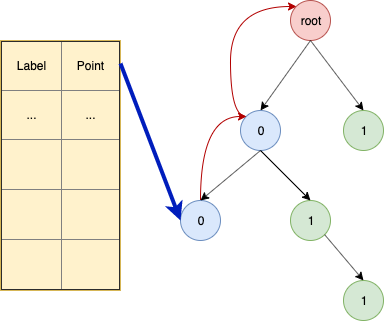# Angora: Efficient Fuzzing by Principled Search

### 0x00 解决路径问题的五种技术

1. 上下文敏感路径覆盖 （像AFL这种都是基于bitmap的edge计数，并不细分edge的上下文）

2. 关于taint analysis 确定 input 和 path constraints之间的映射。

3. 梯度下降算法 作用在 input mutate(ion)

4. input的结构推导 （shape => 结构， type => 结构里面字段）

5. input长度的探索方法

### 0x01 Something interesting

\left\{ \begin{array} \ (l_{prev},l_{cur},context) \\ h(stack) \end{array} \right.

10: L3:true;L4:false;L3:false;L10:true;
01: L3:false:L10:true;L3:true:L4:true;L5:(true|false);

void f(int x){
...
}

void main(){
if(input()=='111'){
f(input());
f(input());
}
}


1. Binary tree：（ b 代表一个位向量， |b| 代表位向量的长度，长度概念应用树里面，就是指结点的高度）每一个位向量 b ,都可以用这个树上的高度为 |b| 的节点 v_b 唯一表示，从根节点rootv_b 路径上的节点依次展开为 b_0,b1,\ldots 。其中的每个结点都一个指向它父结点的指针，这样可以从 v_b 开始顺着树回溯遍历得到完整 b

2. Look-up table: 表里存储着 t_x \rightarrow v_b , 即保存着指向 Binary tree里面结点的指针。t_x 插入操作
1. 去掉 v_b 结尾所有的0。
2. 遵循Binary tree策略，用 b 去遍历Binary tree，如果 b_i=0 ,则follow左子结点，反之亦然，如果子结点不存在则创建结点。
3. 把 t_x 存储到树上最后一个visited的结点。

S= 2n+2(n-1)+4(n-2)+\cdots+(2^{log_2l-1})(log_2-1) \\ S= nl-\sum_{n=1}^{log_2l-1} n2^n \\ S= nl - (l*logl) ,(\sum_{n=1}^{m} n2^n = (n-1)2^{m+1}+2)

Maybe i am wrong,who know!

a \odot b = ?

if(a&b){}

//transform
if(a){
if(b){

}
}


\nabla f(\mathbf{x}) = \bigg(\frac{\partial f}{\partial x_{1}}(\mathbf{x}), \cdots, \frac{\partial f}{\partial x_{n}}(\mathbf{x})\bigg)

\frac{\partial f(\mathbf{X})}{\partial x_i} = \frac{f(\mathbf{X}+\delta v_i)-f(\mathbf{X})}{\delta}

\delta 是一个极小的正数（e.g. ,1）， v_i 表示 i 方向上的单位向量。为了计算每个方向上的偏导数，需要跑两次input,第一次使用original input ，第二次使用经过变换preturbed input X+\delta v_i ，这里有一个非常重要的问题，有可能第二次使用preturbed input的时候，导致 f 所指的二元运算所在程序位置变成了unreachable，这个时候会导致无法计算或者计算出来的结果是毫无意义的。原文在这里没有细谈，而是一笔带过，但是在Matryoshka中在这里提到一种解决方法，有兴趣的同学可以去看看。

f(\mathbf{X}) 最后是否能收敛到理想状态的下(小于0或者小于等于0) ，文中提到了三个点：

• f(\mathbf{X}) 是一个单调函数或者凸函数。
• 局部最小值满足约束。
• 如果局部最小值不满足于约束，Angora将会随机的使用其他的 \mathbf{X}' ，直到局部最小值满足约束。

Angora里面的primitive type分为1，2，4，8bytes。它们之前可能会出现矛盾情况（e.g. 相同连续位置，被fetch一次8bytes，同时也被fetch一次4bytes），这种情况下，取最短的type。类型推断的依据使用其上下文的语义所决定的，比如某个指令操作对象是signed int,那么对应的type也是signed int。注意其整个过程都是在动态的污点分析中完成的。

Angora这里特别地采取了关于输入长度的判断策略，通过关联read-like函数和read 系统调用，对read-like函数传参判定对应的输入offset(taint相关), 还会特殊标记它们的返回值，如果它们的返回值流到了路径条件上，且没有满足路径条件，Angora会尝试增加输入长度。这里我觉得这个策略并不会普遍适用于一般的程序输入，只能说一种有趣的尝试。